GCSE Maths Geometry and Measure

Congruence And Similarity

# Congruence And Similarity

Here we will learn about congruence and similarity, including what congruent shapes are and what similar shapes are. This will extend to congruent triangles.

There are also congruence and similarity worksheets based on Edexcel, AQA and OCR exam questions, along with further guidance on where to go next if you’re still stuck.

## What is congruence and similarity?

Congruence and similarity are mathematical terms used to describe how two or more shapes are related to each other.

### What is congruence and similarity?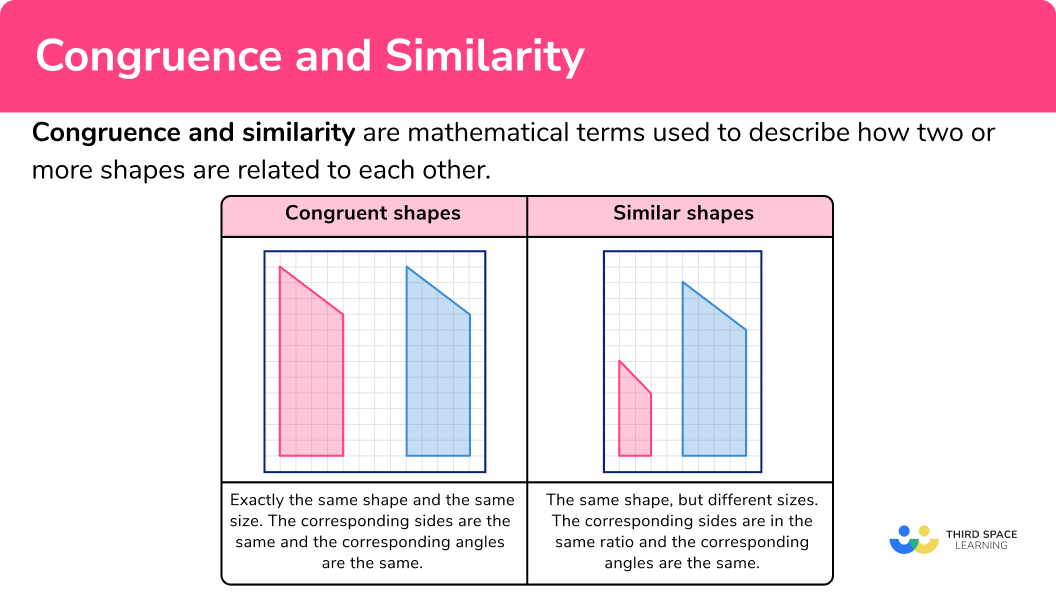• Congruent shapes

Congruent shapes are exactly the same shape and the same size.

The corresponding sides are the same and the corresponding angles are the same. Shapes can be congruent if they have been reflected or rotated.

For example, these polygons are congruent.

Step-by-step guide: Congruent shapes

• Congruent triangles

Congruent shapes can be extended to congruent triangles. There are 4 special conditions to help us recognise congruent triangles. These conditions help us prove triangle congruence.

The 4 reasons are

SSS (side-side-side),

RHS (right-angled triangle, hypotenuse and a side the same),

ASA or AAS (angle-side-angle or angle-angle-side),

SAS (side-angle-side, two sides and the included angle).

Step-by-step guide: Congruent triangles

• Similar shapes

Similar shapes are the same shape but they have different sizes. The corresponding sides are in the same ratio and the corresponding angles are the same.

If we know that 2 shapes are mathematically similar we can work out any missing sides using the ratio, or scale factor.

For example, here are 2 similar triangles.

The ratio of the sides is 4:12=1:3, the scale factor is 12 \div 4=3. We can use either of these to work out the size of the required length.

Therefore x=3\times 7=21.

Step-by-step guide: Similar shapes

## How to use congruence and similarity

In order to answer congruence and similarity questions there are three key skills you need to know how to do. Each of which is explained in the examples below. These skills are,

• Recognising congruent shapes
• Recognising and proving congruent triangles
• Recognising and using similar shapes

### Explain how to use congruence and similarity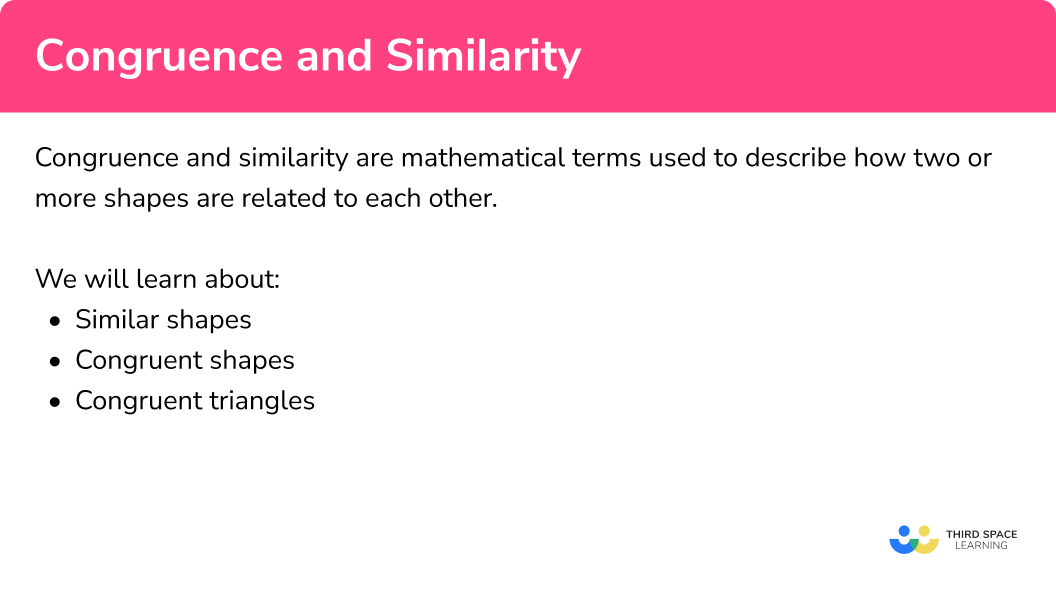## Congruence and similarity examples

### Example 1: congruent shapes

Are these 2D shapes congruent?

1. Check the type of 2D shape.

Both shapes are rectangles.

2Check the corresponding angles and corresponding sides.

All the angles are 90^{\circ}.

The short sides on both rectangles are 3.

The long sides on both rectangles are 5.

3State if the shapes are congruent or not.

The shapes are the same shape and the same size – they are congruent shapes.

### Example 2: recognise congruent triangles

Decide whether this pair of triangles are congruent. If they are congruent, state why.

Check the corresponding angles and corresponding sides.

Decide if the shapes are congruent or not.

If the triangles are congruent, which congruence condition fits the pair of triangles.

### Example 3: prove congruent triangles (higher)

Prove that triangle ABC is congruent to triangle XYZ.

Pair up the corresponding sides.

Pair up the corresponding angles.

State which congruence condition fits the pair of triangles.

### Example 4: finding a missing length using similar shapes

Here are two similar shapes. Find the length PQ.

Decide which sides are pairs of corresponding sides.

Find the scale factor.

Use the scale factor to find the missing length.

### Example 5: finding a missing area using similar shapes (higher)

These two figures are similar.

The area of shape A is 10cm^{2}.

Find the area of shape B.

Find the scale factor.

Use the scale factor to find the missing value.

### Example 6: finding a missing volume using similar shapes (higher)

These two shapes are similar.

The volume of shape A is 120cm^{3}.

Find the volume of shape B.

Find the scale factor.

Use the scale factor to find the missing value.

### Common misconceptions

• Shapes can be congruent but in different orientations

The second shape may be in a different orientation to the first shape. The shapes can still be congruent. Perhaps use tracing paper to help you check.

• Shapes can be congruent but mirror images

The second shape may be a mirror image of the first shape. The shapes can still be congruent. Perhaps use tracing paper to help you check.

• In most diagrams the diagrams are NOT drawn to scale

Questions about congruent shapes are often on grids. But sometimes diagrams may have shapes which are NOT drawn to scale. So use the measurements given, rather than measuring for yourself.

• Scaling up or down

If you are finding a missing length in the larger shape you can multiply by the scale factor. The scale factor will be a number greater than 1.

If you are finding a missing length in the smaller shape you can multiply by the scale factor, but the scale factor will be a number between 0 and 1.

### Practice congruence and similarity questions

1. Which of these shapes is congruent to shape X?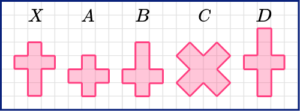ABCDShape B is the same as the original shape, but is upside down.

2. Here is a pair of congruent triangles. Which congruence condition is satisfied?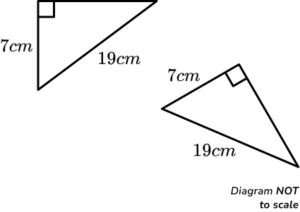ASASASSSSRHSThe triangles are right-angled triangles. They have the same hypotenuse and the same short side.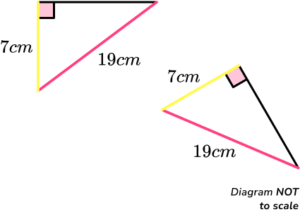3. Prove that triangle ABD is congruent to triangle DEF.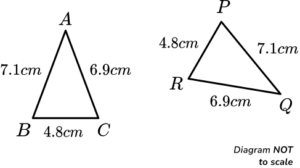SSS

Because

\begin{aligned} \\ AB &= PQ \\ AC &= RQ\\ BC &= PR \end{aligned}SSS

Because

\begin{aligned} \\ AB &= RQ \\ AC &= PQ \\ BC &= PR \end{aligned}SSS

Because

\begin{aligned} \\ AB &= PR \\ AC &= RQ\\ BC &= PQ \end{aligned}SSS

Because

\begin{aligned} \\ AB &= PR \\ BC &= PQ\\ AC &= RQ \end{aligned}The corresponding sides need to be paired up.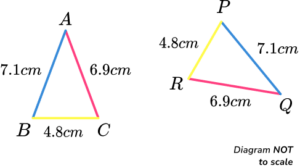4. These parallelograms are similar. Find the value of x.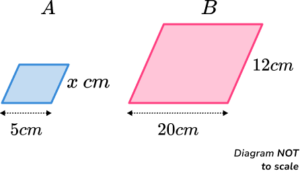x=3.5x=3x=4x=4.5The ratio of the bases is 5:20 which simplifies to 1:4.

The scale factor of enlargement is 4. But we will need to divide by 4 as we are finding a length on the smaller shape.

x=12\div4=3

5. Here are two similar shapes. The area of shape A is 60 \ cm^{2}. Find the area of shape B.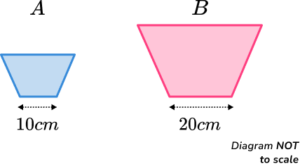240cm^2120cm^2180cm^2200cm^2The ratio of the bases is 10:20 which simplifies to 1:2.

The scale factor of enlargement is 2. But we are finding the area, so we need to multiply the area of shape A by 2^2 or 4.

60\times 2^2=60\times 4=240

So the area of shape B is 240cm^{2}.

6. Here are two similar shapes. The volume of shape B is 35840cm^{3}. Find the volume of shape A.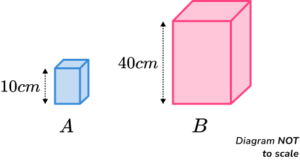4480cm^3560cm^38960cm^32240cm^3The ratio of the bases is 10:40 which simplifies to 1:4.

The scale factor of enlargement is 4. But we are finding the volume, so we need to use 4^3 or 64. Since we are finding the volume of the smaller shape we divide by the scale factor.

35840\div 4^3=35840\div 64=560

So the volume of shape A is 560cm^{3}.

### Congruence and similarity GCSE questions

1. Which shapes are congruent to shape X?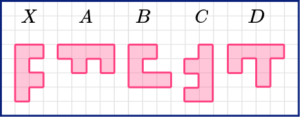(2 marks)

A and C

(2)

2. Rectangles ABCD and PQRS are similar.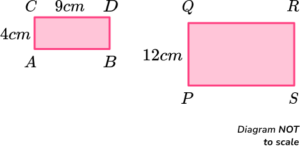\begin{aligned} &AC = 4 \ cm \\ &CD = 9 \ cm \\ &PQ = 12 \ cm \end{aligned}

Work out the length QR.

(2 marks)

9 \times \frac{12}{4}

(1)

27cm

(1)

3. ACD and BCE are straight lines.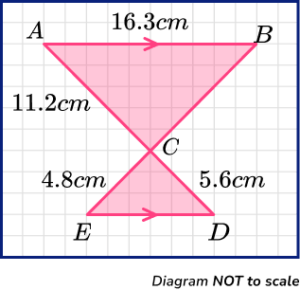AB and DE are parallel.

\begin{aligned} &AB = 16.3 \ cm \\ &AC = 11.2 \ cm \\ &CD = 5.6 \ cm \\ &CE = 4.8 \ cm \end{aligned}

(a) Calculate the length BC.

(b) Calculate the length DE.

(4 marks)

(a)

4.8 \times \frac{11.2}{5.6}

(1)

9.6cm

(1)

(b)

16.3 \div 2

(1)

8.15cm

(1)

## Learning checklist

You have now learned how to:

• Recognise congruent shapes
• Identify congruent shapes
• Identify congruent triangles and their conditions of congruence
• Prove two triangles are congruent (higher)
• Find missing lengths of similar shape
• Find areas and volumes of similar shapes (higher)

## Still stuck?

Prepare your KS4 students for maths GCSEs success with Third Space Learning. Weekly online one to one GCSE maths revision lessons delivered by expert maths tutors.

Find out more about our GCSE maths tuition programme.